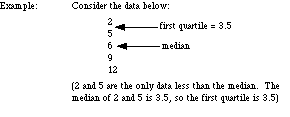# first quartile

First Quartile
Low Quartile
Lower Quartile
Q1

For a set of data, a number for which 25% of the data is less than that number. The first quartile is the same as the median of the data which are less than the overall median. Same as the 25th percentile.